Test: Bilinear Transformations

# Test: Bilinear Transformations

Test Description

## 10 Questions MCQ Test Signals and Systems | Test: Bilinear Transformations

Test: Bilinear Transformations for Electrical Engineering (EE) 2023 is part of Signals and Systems preparation. The Test: Bilinear Transformations questions and answers have been prepared according to the Electrical Engineering (EE) exam syllabus.The Test: Bilinear Transformations MCQs are made for Electrical Engineering (EE) 2023 Exam. Find important definitions, questions, notes, meanings, examples, exercises, MCQs and online tests for Test: Bilinear Transformations below.
Solutions of Test: Bilinear Transformations questions in English are available as part of our Signals and Systems for Electrical Engineering (EE) & Test: Bilinear Transformations solutions in Hindi for Signals and Systems course. Download more important topics, notes, lectures and mock test series for Electrical Engineering (EE) Exam by signing up for free. Attempt Test: Bilinear Transformations | 10 questions in 10 minutes | Mock test for Electrical Engineering (EE) preparation | Free important questions MCQ to study Signals and Systems for Electrical Engineering (EE) Exam | Download free PDF with solutions
 1 Crore+ students have signed up on EduRev. Have you?
Test: Bilinear Transformations - Question 1

### Bilinear Transformation is used for transforming an analog filter to a digital filter.

Detailed Solution for Test: Bilinear Transformations - Question 1

Explanation: The bilinear transformation can be regarded as a correction of the backward difference method. The bilinear transformation is used for transforming an analog filter to a digital filter.

Test: Bilinear Transformations - Question 2

### Which of the following rule is used in the bilinear transformation?

Detailed Solution for Test: Bilinear Transformations - Question 2

Explanation: Bilinear transformation uses trapezoidal rule for integrating a continuous time function.

Test: Bilinear Transformations - Question 3

### Which of the following substitution is done in Bilinear transformations?

Detailed Solution for Test: Bilinear Transformations - Question 3

Explanation: In bilinear transformation of an analog filter to digital filter, using the trapezoidal rule, the substitution for ‘s’ is given as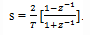Test: Bilinear Transformations - Question 4

What is the value of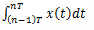according to trapezoidal rule?

Detailed Solution for Test: Bilinear Transformations - Question 4

Explanation: The given integral is approximated by the trapezoidal rule. This rule states that if T is small, the area (integral) can be approximated by the mean height of x(t) between the two limits and then multiplying by the width. That is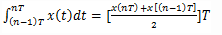Test: Bilinear Transformations - Question 5

What is the value of y(n)-y(n-1) in terms of input x(n)?

Detailed Solution for Test: Bilinear Transformations - Question 5

Explanation: We know that the derivate equation is
dy(t)/dt=x(t)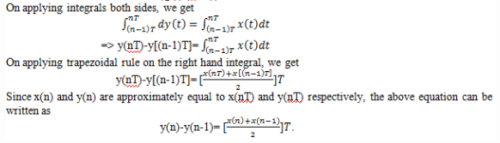Test: Bilinear Transformations - Question 6

What is the expression for system function in z-domain?

Detailed Solution for Test: Bilinear Transformations - Question 6

Explanation: We know that
y(n)-y(n-1)= [(x(n)+x(n-1))/2]T
Taking z-transform of the above equation gives
=>Y(z)[1-z-1]=([1+z-1]/2).TX(z)
=>H(z)=Y(z)/X(z)= T/2[(1+z-1)/(1-z1 )].

Test: Bilinear Transformations - Question 7

In bilinear transformation, the left-half s-plane is mapped to which of the following in the z-domain?

Detailed Solution for Test: Bilinear Transformations - Question 7

Explanation: In bilinear transformation, the z to s transformation is given by the expression
z=[1+(T/2)s]/[1-(T/2)s].
Thus unlike the backward difference method, the left-half s-plane is now mapped entirely inside the unit circle, |z|=1, rather than to a part of it.

Test: Bilinear Transformations - Question 8

The equation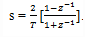is a true frequency-to-frequency transformation.

Detailed Solution for Test: Bilinear Transformations - Question 8

Explanation: Unlike the backward difference method, the left-half s-plane is now mapped entirely inside the unit circle, |z|=1, rather than to a part of it. Also, the imaginary axis is mapped to the unit circle. Therefore, equation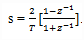is a true frequency-to-frequency transformation.

Test: Bilinear Transformations - Question 9

If s=σ+jΩ and z=re, then what is the condition on σ if r<1?

Detailed Solution for Test: Bilinear Transformations - Question 9

Explanation: We know that if =σ+jΩ and z=re, then by substituting the values in the below expression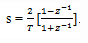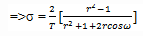When r<1 => σ < 0.

Test: Bilinear Transformations - Question 10

If s=σ+jΩ and z=re and r=1, then which of the following inference is correct?

Detailed Solution for Test: Bilinear Transformations - Question 10

Explanation: We know that if =σ+jΩ and z=re, then by substituting the values in the below expression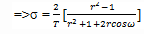=>σ = 2/T[(r2-1)/(r2+1+2rcosω)] When r=1 => σ = 0.
This shows that the imaginary axis in the s-domain is mapped to the circle of unit radius centered at z=0 in the z-domain.

## Signals and Systems

32 videos|76 docs|63 tests
 Use Code STAYHOME200 and get INR 200 additional OFF Use Coupon Code
Information about Test: Bilinear Transformations Page
In this test you can find the Exam questions for Test: Bilinear Transformations solved & explained in the simplest way possible. Besides giving Questions and answers for Test: Bilinear Transformations, EduRev gives you an ample number of Online tests for practice

## Signals and Systems

32 videos|76 docs|63 tests